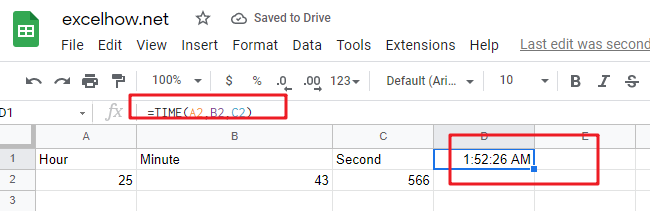# ExcelHow

This post will guide you how to use Google Sheets TIME function with syntax and examples.

## Description

The Google Sheets TIME function converts an hour, minute and second into a time.

The TIME function can be used to create a time with individual hour, minute and second components in google sheets. The purpose of this function is to create a time with the specified hours, minutes and seconds and it also will return a decimal number representing a particular time in google sheets.

The TIME function is a build-in function in Google Sheets and it is categorized as a Date function.

## Syntax

The syntax of the TIME function is as below:

= TIME(hour, minute, second)

Where the TIME function arguments are:

• Hour -This is a required argument. A number from 0 (zero) to 32767 representing the hour. If the value is greater than 23 and then it will be divided by 24 and the remainder will be treated as the hour value. For example, the TIME(28,0,0) is equal to TIME(4,0,0).
• Minute – This is a required argument. A number from 0 to 32767 representing the minute. If the minute value is greater than 59 and it will be converted to hours and minutes (it means that every 60 minutes will add one hour to hour value). For example, TIME(1,75,0) = TIME(2,15,0).
• Second – This is a required argument. A number from 0 to 32767 representing the second. If the second value is greater than 59 and it will be converted to hours, minutes, and seconds (it means that every 60 seconds will add 1 minute to minute value). For example, TIME(1,20,75) = TIME(1,21,15) .

Note:

• All of arguments must be numbers and if a string or a cell reference that containing a text string is provided, and TIME function returns #VALUE! Error message.
• TIME function will truncate decimal values of input into the function, for example, an hour component value of 10.58 will be recognized as 10.

## Google Sheets TIME Function Examples

The below examples will show you how to use google sheets TIME function to create a decimal number representing a particular time.

#1 =TIME(A2,B2,C2)